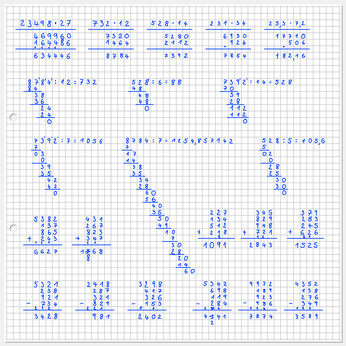# Graph paper is beneficial for all math students!When you are shopping for school supplies, think about an extra journal or package of three ring binder paper: quad lined paper is the first and most economical help for your struggling Math student. A small minority of students gets visually confused by the squares on the page. So it is always best to search for paper that is very lightly printed with ‘unobtrusive’ squares: the emphasis should be on what your student is writing and drawing not on the grid.

Neatly lining up calculations prevents errors. Quad paper can also help illustrate many concepts that are more complicated to explain in words but are easily seen on paper, such as multiplication, area and perimeter, for making graphs, and drawing congruent shapes, when showing transformations in size, slides, flips, and rotations and many more.Children learn by doing

Research in education tells us over and over that hands on activities have more impact than verbal or written explanations alone. Simple manipulatives and pictures are used as key elements to illustrate math concepts.

Download our free guidelines and templates for games, outright playful and more serious activities to develop the math centers in your children’s brain. There are sample activities to develop number sense, visuo-spatial activities and templates. Have fun!

Please register for free, to give us an idea if activities are useful as a guidance to expand the website in the future. We never share your information with any other organization, see our Terms and Conditions – Privacy Policy.

 Number sense activities Math concept Rules of Thumb General approach for teaching your child Number walk and Bedtime book Learning number words, counting, one to one relationship, starting with the numerals Take a guess before you count Subitizing, small quantity estimation, quantity comparison Learning to count on the kitchen tiles Counting up and counting down, numerals First, Second, and Third place Play a game to learn the cardinal numbers Number cards counting and sorting activity  NEW Counting by ones and tens, and sorting by tens Number-line addition and subtraction Number-line, distance, counting, skip counting, addition, subtraction On the Double Introducing the concept of multiplication by doubling Conquer the Multiplication Tables Basic understanding of the multiplication tables till ten Help with fractions Help your child to grasp the concept of fractions Understanding fractions and Fractions Booklet Explanation how to make the most of the Fractions Booklet Fractions Booklet My fractions book: whole and parts, add and subtract like parts Fraction Notation activities and cards NEW Understanding Fraction notation and concepts Cross out cross-multiplication Prevent confusion with cross-multiplication by showing all steps Visuo-spatial activities Math concept Symmetry activity: looking left and right Symmetry drawings: sizes and shapes, line of symmetry, symmetrical shape Ruler number sense activity NEW Estimating size of numbers Thinking about Area by folding Fold to divide an area in similar parts, area is width times length Pegboards Copy patterns and shapes Patterns from concrete to abstract From patterns with concrete objects to number patterns Templates Math concept Counting place-mat Counting to five Number-bonds place-mat Number-bonds to ten Number cards Color coded number cards 1 – 100 Base ten template Ones, tens, hundreds Domino Doubles cards Learning the Doubles from 0 – 9